### Home > MC2 > Chapter 7 > Lesson 7.2.1 > Problem7-71

7-71.
1.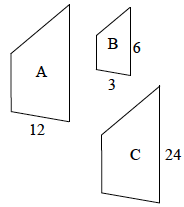Use the similar shapes below to answer the questions below. Homework Help ✎

1. What is the scale factor from shape A to shape B?

2. What is the scale factor from shape B to shape C?

3. How are these scale factors related?

4. What do you know about shape A and shape C?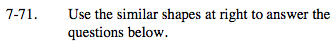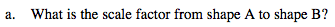What is the factor between 12 and 3?

Divide by 4.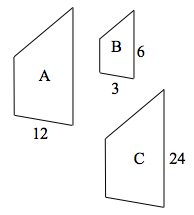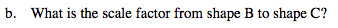What is the factor between 6 and 24?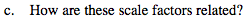Do you notice any similar changes from shapes A to B and B to C? Do they have similar factors?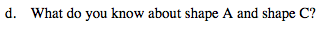They are congruent.